# Lookup函數最經典的14種用法

4.8
(58)

## 1、普通查找（已排序）

【例】查找香蕉的數量
=LOOKUP(D2,A:B)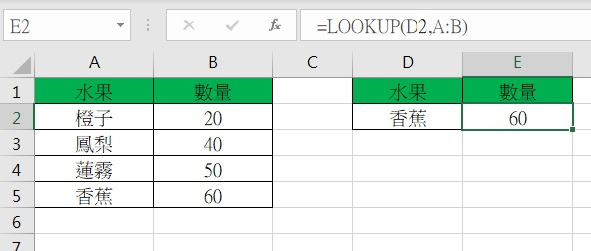## 2、普通查找（未排序）

A列未排序的表格，就需要用0/的方法完成查找。
【例】查找香蕉的數量
=LOOKUP(1,0/(A2:A6=D2),B2:B6)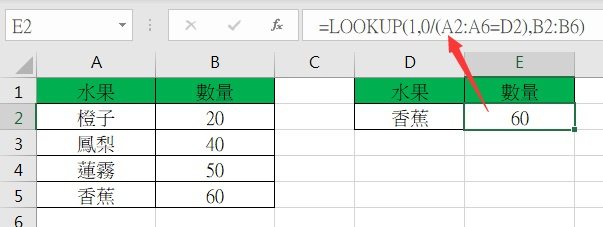## 3、查找最後一個非數字內容

【例】查找D列最後一個非數字字符
=LOOKUP(“座”,A:A)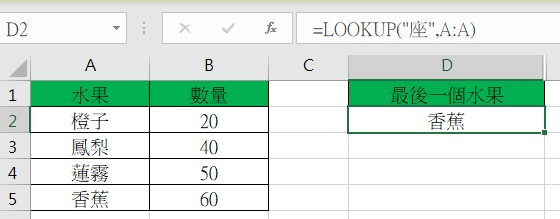## 4、查找最後一個數字

【例】查找B列最後一個數字
=LOOKUP(9E+307,B:B)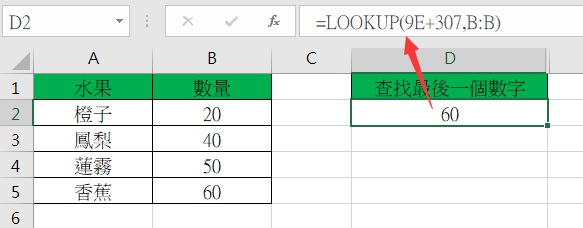## 5、查找最後一個非空內容

【例】查找A列最後一個非空內容
=LOOKUP(1,0/(A1:A28<>””),A1:A28)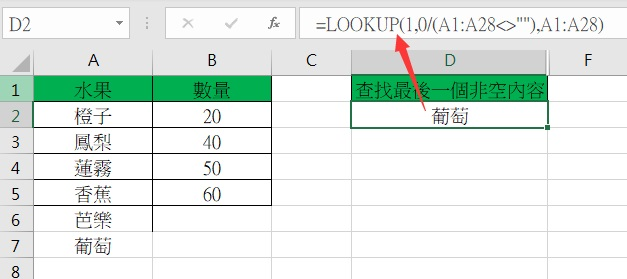## 6、根據條件查找最後一個

【例】查找橙子最後一次數量
=LOOKUP(1,0/(A2:A7=D2),B2:B7)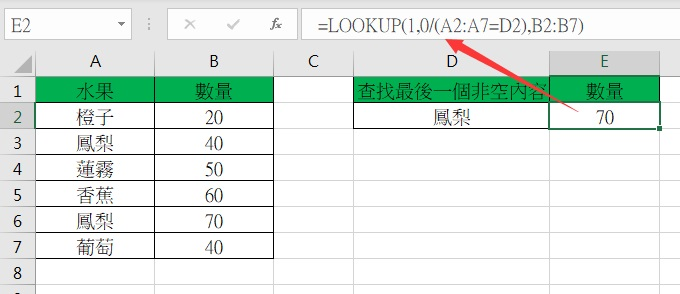## 7、區間查找

【例】根據D2的銷量查找對應區間提成
=LOOKUP(D2,A:B)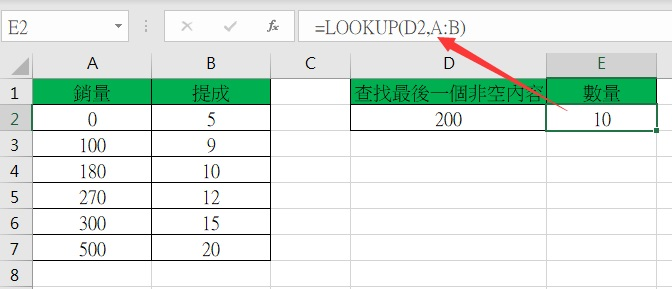## 8、多條件查找

【例】查找長江店的橙子數量
=LOOKUP(1,0/((B2:B7=F2)*(A2:A7=E2)),C2:C7)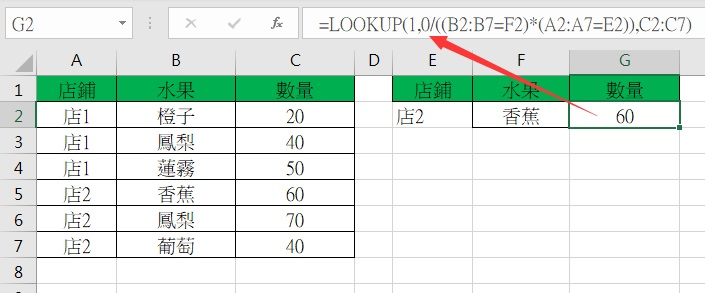## 9、根據簡稱查全稱

【例】如下圖所示，要求根據提供的城市從上表中查找該市名的第2列的值。
=LOOKUP(9^9,FIND(A7,A2:A4),B2:B4)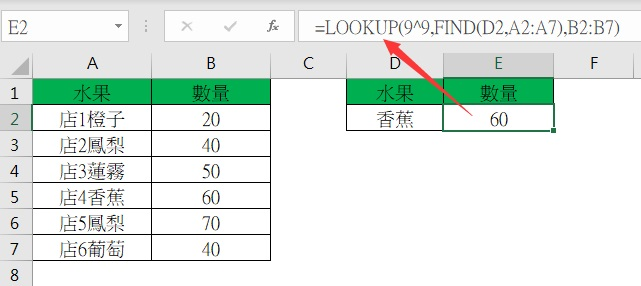## 10、根據全稱查簡稱

【例】如下圖所示，要求根據地址從上表中查找所在城市的提成。
=lookup(9^9.find(A\$3:A\$6,A10),B\$3:B\$6)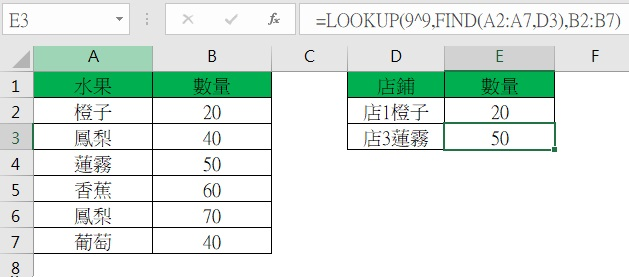## 11、包含查找

【例】如下圖所示的A列，是包括車類別的明細車型，現需要在B列把車類別提取出來。 （車類別有四種：捷達，速騰,邁騰，高爾夫）
=LOOKUP(9^9,FIND({“捷達”,”速騰”,”邁騰”,”高爾夫”},A2),{“捷達”,”速騰”,”邁騰”,”高爾夫”})## 12、數字提取（數字在開頭）

【例】A1的值為 123.45ABC，截取結果：123.45
=LOOKUP(9^9,LEFT( A1,ROW(1:9))*1)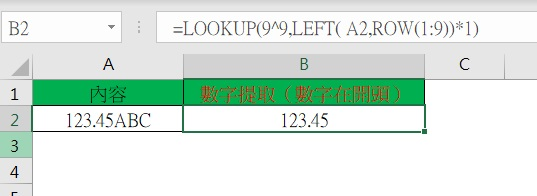## 13、數字提取（數字在結尾頭）

【例】A1的值為 ABC123.45截取結果：123.45
=LOOKUP(9^9,RIGHT(A1,ROW(1:9))*1)## 14、數字提取（數字在任意位置）

【例】A1的值為 ABC123.45FE截取結果：123.45
{=LOOKUP(9^9,MID(A1,MATCH(1,MID(A1,ROW(1:9),1)^0,0),ROW(1:9))*1)}
lookup函數的經典用法差不多整理齊了，學會這些工作中已沒有難倒你的查找公式了。一定一定要收藏好哦

### 網友評論3步驟救回500多種被刪除、格式化或丟失的檔案、照片、視訊、音訊，以及各種狀況丟失的資料檔案。